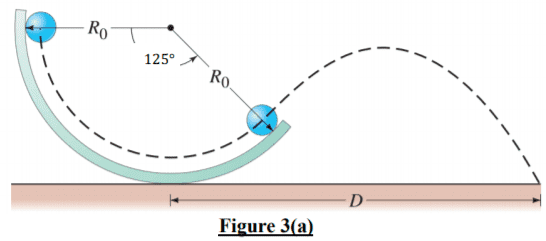# Conservation of energy, centripetal acceleration, kinematics

wcjy
Homework Statement:
A small sphere of radius r_0 = 1.5 cm rolls without slipping on the track which is fixed on the
ground as shown in Figure 3(a). The radius of the track is R_0 = 26 cm. The sphere is released
from rest at height R_0 above the bottom of the track and rolls without slipping down the track.

(a) What is the angular velocity of the sphere when it leaves the track after passing through
an angle 125° as shown in Figure 3(a)

(b) What is the net acceleration of the sphere the moment before it leaves the track?

(c) At what distance D from the base of the track will the sphere hit the ground?
Relevant Equations:
Conservation of Energy
a_c = v^2 / r(a) Using COE,
$$mgh = 0.5mv^2 + 0.5I\omega^2$$
I solved it, where $$\omega = 112 rad/s$$

(b) This is the part where I have question or problem.
I saw my course mate working and he start of with finding centripetal acceleration.
$$a_c = \frac{v^2}{r} = \frac{(r_0\omega)^2}{R_0}$$

Why isn't it:
$$a_c = R_0\omega^2$$

Homework Helper
Gold Member
2022 Award
Don't confuse the angular velocity of the sphere about its own centre with the angular velocity of the centre about the centre of curvature of the track.

•wcjy
Homework Helper
Gold Member
(b) This is the part where I have question or problem.
I saw my course mate working and he start of with finding centripetal acceleration.
$$a_c = \frac{v^2}{r} = \frac{(r_0\omega)^2}{R_0}$$

Why isn't it:
$$a_c = R_0\omega^2$$
Neither is quite right. Although the approach by your course mate is closer.

Centripetal acceleration is
$$a = \frac{\left( \mathrm{speed \ of \ object} \right)^2}{\mathrm{radius \ of \ curvature \ of \ the \ object's \ path}}$$

The speed, with respect to the ground, of the ball is $r_0 \omega$ (where $r_0$ is the radius of the ball, and $\omega$ is the angular velocity of the ball around the ball's own center).

$R_0$ is the radius of curvature of the track, but that's not quite the same as the radius of curvature of the path of the ball as it rolls on the track. It's close, but not quite. Look at the diagram carefully. The radius of curvature of the ball's path on the track is what you are looking for. (Hint: the ball's radius is not zero.)

•wcjy
wcjy
Thanks for all your explanation. I think i get it. will be attempting the rest of the question and see if i face any difficulties. Thank You So Much!